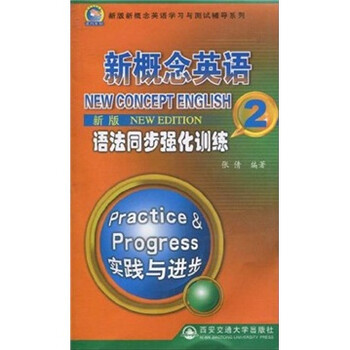• 纸质书
• 全部
• 电子书

I  S  B  N：•0.00

• 配送范围:
全国(除港澳台地区)
• ISBN：
9787560532912
• 作      者：
张倩编著
• 出 版 社 ：
西安交通大学出版社
• 出版日期：
2010《新概念英语语法同步强化训练2(实践与进步)(新版)》主要特点：紧贴《新概念英语》，帮助学习者从英语学习的各个纬度提高英语水平。内容精炼，有讲有练，以课为单位。为每篇课文精心设计练习题目。题型多样，兼顾能力训练和考试要求。版式简洁，图书小巧，便于携带，方便学习者随时随地学习英语。

《新概念英语语法同步强化训练2(实践与进步)(新版)》是一部风靡全球的经典英语教程，它以其严密的体系性、严谨的科学性、精湛的实用性、浓郁的趣味性受到了世界各地英语学习者的青睐，也在中国的英语学习者中赢得了无可比拟的盛誉，成为英语学习者的首选教材。

1.现在完成时和一般过去时都表示过去发生的动作，但现在完成时强调这一动作与现在的关系，如对现在产生的结果、影响等，而一般过去时则单纯表示发生在过去的动作。如：我来为你介绍一下史密斯先生。（强调的是现在已经认得了，所以无需介绍），前几天我在街上见过他。（仅强调过去见过这一事实）。
2.一般过去时常与具体的时间状语连用，而现在完成时通常与模糊的时间状语连用，或无时间状语（参见第四课）。如：昨天一只鸽子把第一封信从平赫斯特带到了索尔伯利。他以前从未哭过。注意：有几个动词和介词的搭配在现在完成时里有各自意义。如：他已经在法国呆了三年了。（现在人还在法国）他去过法国。（现在人已经回来了）他去了法国。（过去去的，现在人还没回来）他去年去了法国。（仅强调去过这个事实）。

Lesson 1
Lesson 2
Lesson 3
Lesson 4
Lesson 5
Lesson 6
Lesson 7
Lesson 8
Lesson 9
Lesson 10
Lesson 11
Lesson 12
Lesson 13
Lesson 14
Lesson 15
Lesson 16
Lesson 17
Lesson 18
Lesson 19
Lesson 20
Lesson 21
Lesson 22
Lesson 23
Lesson 24

Lesson 25
Lesson 26
Lesson 27
Lesson 28
Lesson 29
Lesson 30
Lesson 31
Lesson 32
Lesson 33
Lesson 34
Lesson 35
Lesson 36
Lesson 37
Lesson 38
Lesson 39
Lesson 40
Lesson 41
Lesson 42
Lesson 43
Lesson 44
Lesson 45
Lesson 46
Lesson 47
Lesson 48

Lesson 49
Lesson 50
Lesson 51
Lesson 52
Lesson 53
Lesson 54
Lesson 55
Lesson 56
Lesson 57
Lesson 58
Lesson 59
Lesson 60
Lesson 61
Lesson 62
Lesson 63
Lesson 64
Lesson 65
Lesson 66
Lesson 67
Lesson 68
Lesson 69
Lesson 70
Lesson 71
Lesson 72

Lesson 73
Lesson 74
Lesson 75
Lesson 76
Lesson 77
Lesson 78
Lesson 79
Lesson 80
Lesson 81
Lesson 82
Lesson 83
Lesson 84
Lesson 85
Lesson 86
Lesson 87
Lesson 88
Lesson 89
Lesson 90
Lesson 91
Lesson 92
Lesson 93
Lesson 94
Lesson 95
Lesson 96